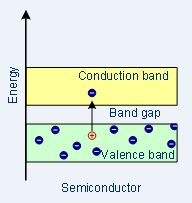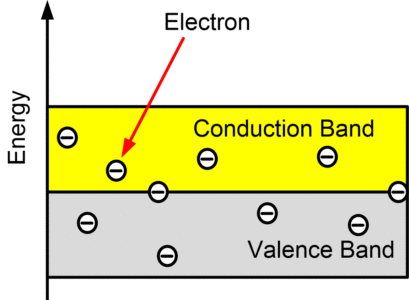Home / Electrical Circuits / Difference between Conductor Semiconductor and Insulator

# Difference between Conductor Semiconductor and Insulator

Want create site? Find Free WordPress Themes and plugins.

This article covers the key differences between Conductor, Semiconductor, and Insulator on the basis of Conductivity, Resistivity, Forbidden Gap, Conduction, Band Structure, Current Flow, Band Overlap, 0 Kelvin Behavior, and Examples. The following table covers the key Differences between Conductor Semiconductor and Insulator.

 Characteristics Conductor Semi-Conductor Insulator Conductivity High Moderate Low Resistivity Low Moderate Very High Forbidden gap No forbidden gap Small forbidden gap Large forbidden gap Temperature coefficient Positive Negative Negative Conduction Large number of electrons for conduction Very small number of electrons for conduction Moderate number of electrons for conduction Conductivity value Very high $\text{1}{{\text{0}}^{\text{-7}}}\text{mho/m}$ Between those of conductors and insulators i.e. $\text{1}{{\text{0}}^{\text{-7}}}\text{mho/m}$ to $\text{1}{{\text{0}}^{\text{-13}}}\text{mho/m}$ Negligible like $\text{1}{{\text{0}}^{\text{-13}}}\text{mho/m}$ Resistivity value Negligible; less than $\text{1}{{\text{0}}^{\text{-5}}}\text{ }\Omega \text{-m}$ Between those of conductors and insulators i.e. $\text{1}{{\text{0}}^{\text{-5}}}\text{ }\Omega \text{-m}$ to $\text{1}{{\text{0}}^{\text{5}}}\text{ }\Omega \text{-m}$ Very high; more than $\text{1}{{\text{0}}^{\text{5}}}\text{ }\Omega \text{-m}$ Band structureCurrent flow Due to free electrons Due to free electrons and holes more than that in insulators Due to free electrons but negligible Number of current carriers at normal temperature Very high Low Negligible Band overlap Both conduction and valence bands are overlapped. Both bands are separated by an energy gap of 1.1eV Both bands are separated by an energy gap of 6eV to 10eV 0 Kelvin Behavior Acts like a superconductor Acts like an insulator Acts like an insulator Formation Formed by metallic bonding Formed by covalent bonding Formed by ionic bonding Valence Electrons One valence electron in the outermost shell Four valence electron in the outermost shell Eight valence electron in the outermost shell Examples Copper, mercury, aluminum, silver Germanium, Silicon Wood, Rubber, Mica, Paper

## Difference between Conductors, Semiconductors, and Insulators on the Basis of Energy Bands

Conductors

In conductive materials, no band gaps exist so electrons move easily using a continuous, partly full conduction band.Semiconductors

In semiconductor materials, the band gap between the conduction band and valence band is smaller and at normal temperature (room temperature), there is enough energy accessible to displace a few electrons from the valence band into the conduction band.
As temperature increases, the conductivity of a semiconductor material increases.Insulator

In insulators, there is a large band gap between the conduction and valence band. The valence band remains full since no movement of electrons occurs and as a result, the conduction band remains empty as well.Did you find apk for android? You can find new Free Android Games and apps.